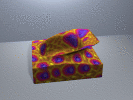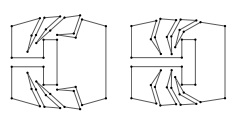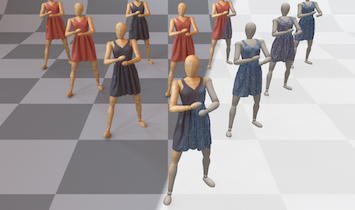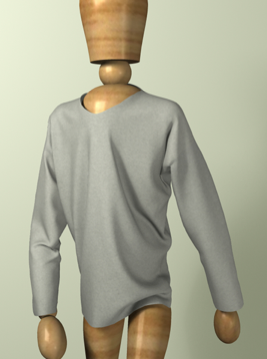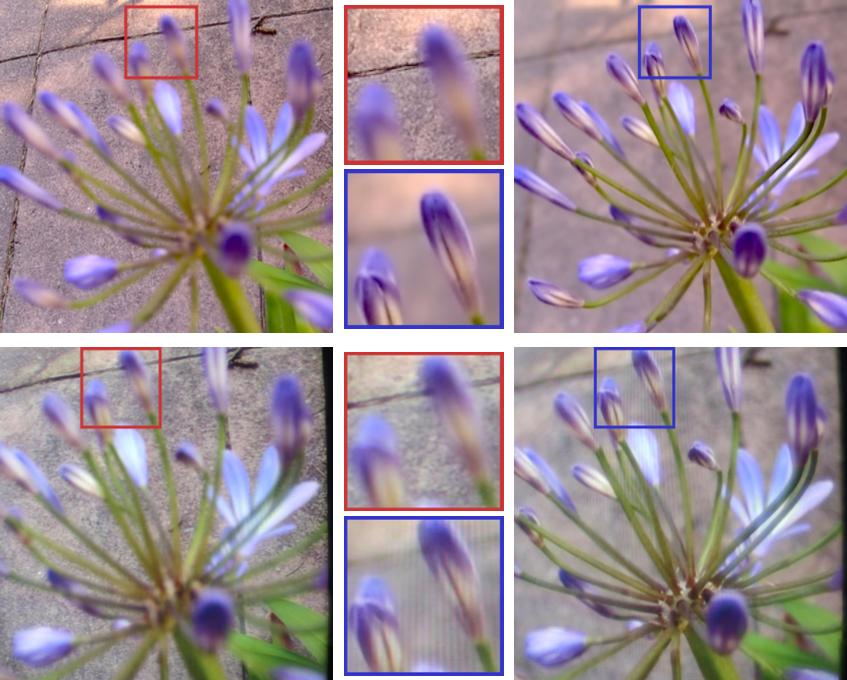# Computer Graphics

## Fast Simulation of Mass-Spring Systems### Abstract

We describe a scheme for time integration of mass-spring systems that makes use of a solver based on block coordinate descent. This scheme provides a fast solution for classical linear (Hookean) springs. We express the widely used implicit Euler method as an energy minimization problem and introduce spring directions as auxiliary unknown variables. The system is globally linear in the node positions, and the non-linear terms involving the directions are strictly local. Because the global linear system does not depend on run-time state, the matrix can be pre-factored, allowing for very fast iterations. Our method converges to the same final result as would be obtained by solving the standard form of implicit Euler using Newton's method. Although the asymptotic convergence of Newton's method is faster than ours, the initial ratio of work to error reduction with our method is much faster than Newton's. For real-time visual applications, where speed and stability are more important than precision, we obtain visually acceptable results at a total cost per timestep that is only a fraction of that required for a single Newton iteration. When higher accuracy is required, our algorithm can be used to compute a good starting point for subsequent Newton's iteration.

### Citation

Tiantian Liu, Adam W. Bargteil, James F. O'Brien, and Ladislav Kavan. "Fast Simulation of Mass-Spring Systems". ACM Transactions on Graphics, 32(6):209:1–7, November 2013. Proceedings of ACM SIGGRAPH Asia 2013, Hong Kong.